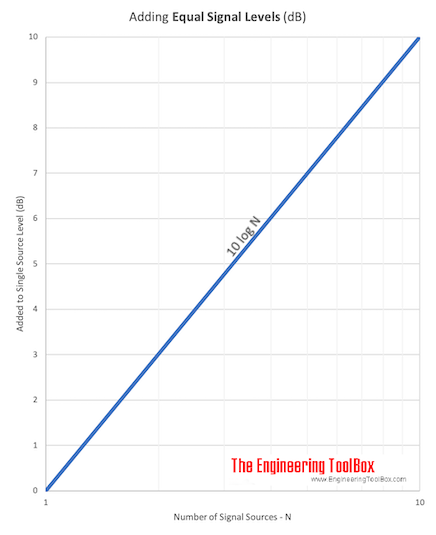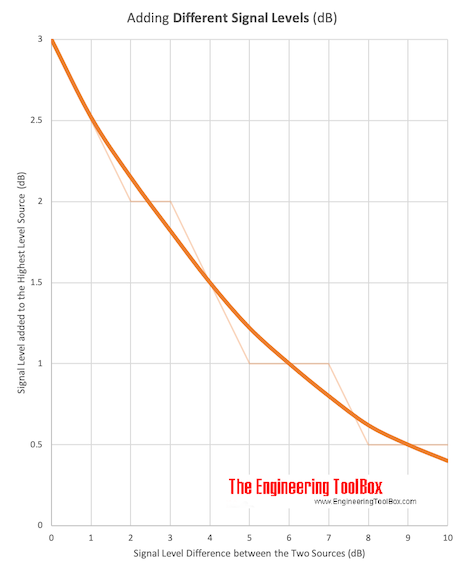Engineering ToolBox - Resources, Tools and Basic Information for Engineering and Design of Technical Applications!

## The logarithmic decibel scale is convenient when adding signal values like sound power, pressure and others from two or more sources.

The decibel (dB) is a logarithmic unit used to express the ratio of two signal values - like power, sound power or pressure, voltage, intensity etc. - where one value is a reference value.

The total signal level in decibel from equal signal sources can be calculated as

Lt =  10 log (n S / Sref)

= 10 log (S / Sref) + 10 log (n)

= Ls + 10 log (n)                                                         (1)

where

Lt = total signal level (dB)

S = signal (signal unit)

Sref = signal reference (signal unit)

n = number of sources

Ls = signal level from each single source (dB)

The signal units depends on the nature of the signal - W for power, Pa for pressure and so on.

Note! -  adding sound pressure levels.

#### Example - Total Sound Power from Two Identical Fans

For sound power it is common to use 10-12 W as the reference sound power. Total sound power from two identical fans each generating 1 W in noise power can be calculated as

Lt =  10 log (2 (1 W) / (1 10-12 W))

= 123 dB

Sound power and sound power level are often used to specify the noise or sound emitted from technical equipment like fans, pumps or other machines. The "sound" measured with microphones or sensors (meters) are sound pressure.

### Adding Equal Signals Units Calculator

S - signal (signal unit)

Sref - signal reference (signal unit)

n - number of signal sources

### Adding Equal Signal Levels (decibels) Calculator

Ls - signal level from single source (decibel, dB)

n - number of signal sources

Adding equal signal sources can be expressed graphicallyDownload and print Adding Signal Level of Equal Sources chart.

Note! Adding two identical sources (doubling the signal) will increase the total signal level with 3 dB (10 log(2)).

Number of
Sources
Increase in
Sound Power Level
(dB)
2 3
3 4.8
4 6
5 7
10 10
15 11.8
20 13

### Adding Signals from Sources with different Strengths

The total signal level from sources with different strengths can be calculated as

Lt =  10 log ((S1 + S2 ... + Sn) / Sref)                                                (2)

#### Example - Total Sound Power from Two different Fans

The total noise power from two fans - one with sound power 1 W and the other with sound power 0.5 W - can be calculated as

Lt =  10 log (((1 W) + (0.5 W)) / (1 10-12 W))

= 122 dB

Adding two signal sources with different levels can be expressed graphically in decibels asSignal Level Difference
between
two Sources
(dB)
Decibels
Highest Signal Level
(dB)
0 3
1 2.5
2 2
3 2
4 1.5
5 1
6 1
7 1
8 0.5
9 0.5
10 0.5
> 10 0

#### Example - Adding Sound Power in Decibels

The sound power from one of the fans in the example above can be calculated as

Ls1 = 10 log((1 W) / (1 10-12 W))

= 120 dB

The sound power from the other fan can be calculated as

Ls2 = 10 log((0.5 W) / (1 10-12 W))

= 117 dB

The difference in decibel is

Ls1 - Ls2

= (120 dB) - (117 dB)

= 3 dB

From the table or diagram above a difference of 3 dB requires that 2 dB must be added to the highest sound pressure source as

Lt = (120 dB) + (2 dB)

= 122 dB

## Related Topics

• Acoustics - Room acoustics and acoustic properties, decibel A, B and C, Noise Rating (NR) curves, sound transmission, sound pressure, sound intensity and sound attenuation.
• Noise and Attenuation - Noise is usually defined as unwanted sound - noise, noise generation, silencers and attenuation in HVAC systems.
• Measurements & Instrumentation - Measurement and instrumentation strategies.

## Engineering ToolBox - SketchUp Extension - Online 3D modeling!

Add standard and customized parametric components - like flange beams, lumbers, piping, stairs and more - to your Sketchup model with the Engineering ToolBox - SketchUp Extension - enabled for use with the amazing, fun and free SketchUp Make and SketchUp Pro .Add the Engineering ToolBox extension to your SketchUp from the SketchUp Pro Sketchup Extension Warehouse!

Translate

## Privacy

We don't collect information from our users. Only emails and answers are saved in our archive. Cookies are only used in the browser to improve user experience.

Some of our calculators and applications let you save application data to your local computer. These applications will - due to browser restrictions - send data between your browser and our server. We don't save this data.

## Citation

• Engineering ToolBox, (2003). Signals - Adding Decibels. [online] Available at: https://www.engineeringtoolbox.com/adding-decibel-d_63.html [Accessed Day Mo. Year].

Modify access date.

. .

#### Scientific Online Calculator3 30